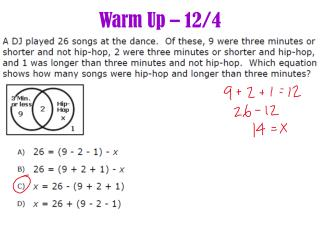DownloadDownload PresentationWarm Up – 12/4

# Warm Up – 12/4

Télécharger la présentation## Warm Up – 12/4

- - - - - - - - - - - - - - - - - - - - - - - - - - - E N D - - - - - - - - - - - - - - - - - - - - - - - - - - -
##### Presentation Transcript

1. Warm Up – 12/4

2. Lesson 22-23: Solving Equations Objective • Students use algebra to solve equations by using techniques of making zero (adding the additive inverse) and making one (multiplying by the multiplicative inverse) to solve for the variable.

3. Example 1: Yoshiro’s New Puppy Yoshiro has a new puppy. She decides to create an enclosure for her puppy in her back yard. The enclosure is in the shape of a hexagon (six-sided polygon) with one pair of opposite sides running the same distance along the length of two parallel flowerbeds. There are two boundaries at one end of the flowerbeds that are 10 ft. and 12 ft., respectively, and at the other end, the two boundaries are 15 ft. and 20 ft., respectively. If the perimeter of the enclosure is 137 ft., what is the length of each side that runs along the flowerbed? Question 1: What is the general shape of the puppy yard? Draw a sketch of the puppy yard.

4. Example 1: Cont… Question 2: Model and solve this equation with a tape diagram. Question 3: Write an equation that would model finding the perimeter of the puppy yard.

5. Example 2: Swim Practice Jenny is on the local swim team for the summer and has swim practice four days per week. The schedule is the same each day. The team swims in the morning and then again for two hours in the evening. If she swims 12 hours per week, how long does she swim each morning? Question 1: What tape diagram would be appropriate for this problem? Question 2: What equation would be appropriate to solve this problem?

6. Exercises 5𝑥 + 4 =19 15𝑥 + 14 = 19 2(𝑥 + 4) = 18

7. Exit Ticket Susan and Bonnie are shopping for school clothes. Susan has \$50 and a coupon for a \$10 discount at a clothing store where each shirt costs \$12. Susan thinks that she can buy 3 shirts, but Bonnie says that Susan can buy 5 shirts. The equations they used to model the problem are listed below. Solve each equation algebraically and determine who is correct and why. Susan’s Equation Bonnie’s Equation 12 𝑛 + 10 = 50 12 𝑛−10 = 50